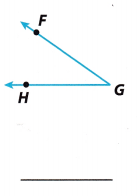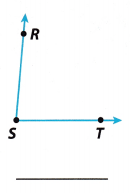# Texas Go Math Grade 8 Module 12 Answer Key Transformations and Congruence

Refer to our Texas Go Math Grade 8 Answer Key Pdf to score good marks in the exams. Test yourself by practicing the problems from Texas Go Math Grade 8 Module 12 Answer Key Transformations and Congruence.

## Texas Go Math Grade 8 Module 12 Answer Key Transformations and Congruence

Find each difference.

Question 1.
5 – (-9) ___________
5 – (-9) = 5 + 9 = 14 (To subtract an integer, add its opposite)

Question 2.
-6 – 8 ___________
(To subtract an integer, add it’s opposite) -6 – 8 = – 6 + (- 8)
(The signs are the same negative, so the sum of integers is negative) = – 14

Question 3.
2 – 9 ___________
(To subtract an integer, add it’s opposite) 2 – 9 = 2 + (- 9)
(The signs are different, so find the difference of the absolute values: 9 – 2 = 7.) = |2| – |-9|
(Use the sign of the number with the greater absolute vaue) = -7

Question 4.
-10 – (-6) ___________
(To subtract an integer, add it’s opposite.) -10 – (- 6) = -10 + 6
(The signs are different, so find the difference of the absolute values: 10 – 6 = 4.) = -(|-10| – |-6|)
(Use the sign of the number with the greater absolute value) = -4

Question 5.
3 – (-11) ___________
3 – (-11) = 3 + 11 = 14 (To subtract an integer, add it’s opposite.)

Question 6.
12 – 7 ___________
(To subtract an integer, add it’s opposite) 12 – 7 = 12 + (- 7)
(The signs are different, so find the difference of the absolute values: 12 – 7 = 5.) = |12| – |7|
(Use the sign of the number with the greater absolute value) = 5

Question 7.
-4 – 11 ___________
(To subtract an integer, add it’s opposite.) -4 – 11 = – 4 + (-11)
(The signs are both negative, so the sum of integers is negative.) = -15

Question 8.
0 – (-12) ___________
0 – (-12) = 0 + 12 = 12 (To subtract an integer, add it’s opposite)

Use a protractor to measure each angle.

Question 9.Use a protractor to determine the angle measure.
The angle measure is 35°.

Question 10.Use a protractor to determine the angle measure.
The angle measure is 130°.

Question 11.Use a protractor to determine the angle measure.
The angle measure is 85°.

Visualize Vocabulary

Use the ✓ words to complete the graphic organizer. You will put one word in each oval.Understand Vocabulary

Match the term on the left to the correct expression on the right.